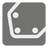Convex Hull
Simple
RU English ES

You are given a list of points on a coordinate plane. We need you find the convex hull formed by these points. The convex hull of a set X of points in the Euclidean plane is the smallest convex set that contains X. For instance: when X is a bounded subset of the plane, the convex hull may be visualized as the shape formed by a rubber band stretched around X. If a point lies on edge, it's included.

The points are presented as a list of coordinates [x, y] in which x and y are integers. The result returns as a sequence of indexes of points in the given list; points lie on the convex hull in clockwise order (see the picture). The sequence starts from the bottom leftmost point. Remember: You should return a list of indexes--not the points themselves.Input: A list of coordinates. Each coordinate is a list of two integers.

Output: The list of indexes of coordinates from the given list.

Example:

```checkio([[7, 6], [8, 4], [7, 2], [3, 2], [1, 6], [1, 8], [4, 9]]) == [4, 5, 6, 0, 1, 2, 3]
checkio([[3, 8], [1, 6], [6, 2], [7, 6], [5, 5], [8, 4], [6, 8]]) == [1, 0, 6, 3, 5, 2]```

How it is used: Convex hulls have practical applications in pattern recognition, image processing, statistics, GIS and static code analysis by abstract interpretation. The concept also serves as a tool and a building block for a number of other computational-geometric algorithms such as the rotating calipers method for computing the width and diameter of a point set.

Precondition:
2 < len(coordinates) < 10
all(0 < x < 10 and 0 < x < 10 for x, y in coordinates)